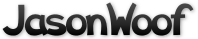Got questions, comments, patches, etc.? Contact Jason Woofenden
 author Joshua Grams Sat, 25 Mar 2006 22:12:55 +0000 (22:12 +0000) committer Joshua Grams Sat, 25 Mar 2006 22:12:55 +0000 (22:12 +0000)
 sprite.c patch | blob | history

index 1a35a6a..447e482 100644 (file)
--- a/sprite.c
+++ b/sprite.c
@@ -336,26 +336,113 @@ sprite_mass(Sprite *s)
else return 0;
}

+/*
+ * BOUNCE THEORY
+ *
+ * ******************  In 1 Dimension  *****************
+ *
+ * For now we will imagine bouncing A and B off each other in 1 dimension (along
+ * a line). We can safely save the other dimension for later.
+ *
+ * A and B are the same weight, and are both traveling 1m/sec, to collide right
+ * at the origin. With perfect bounciness, their full momentum is reversed.
+ *
+ * If we cut the weight of A down by half, then the center of our colision will
+ * drift towards A (the speeds of A and B are not simply reversed as in our last
+ * example.) However, there is always a place between A and B on the line (I'll
+ * call it x) such that the speeds of A and B relative to x, are simply
+ * reversed. Thus we can find the new speed for A like so:
+ *
+ *     new A = x -(A - x)
+ *
+ *     new B = x -(B - x)
+ *
+ * or, simply:
+ *
+ *     new A = 2x - A
+ *
+ *     new B = 2x - B
+ *
+ *
+ * this point x is the sort of center of momentum. If, instead of bouncing, A
+ * and B just globbed together, x would be center of the new glob.
+ *
+ * x is the point where there's an equal amount of force coming in from both
+ * sides. ie the weighted average of the speeds of A and B.
+ *
+ * average force = (A force + B force) / total mass
+ *
+ * x.speed = (a.speed * a.mass + b.speed * b.mass) / (a.mass + b.mas)
+ *
+ * then we apply the formula above for calculating the new A and B.
+ *
+ *
+ *
+ *
+ * ******************  In 2 Dimensions  *****************
+ *
+ * OK, that's how we do it in 1D. Now we need to deal with 2D.
+ *
+ * Imagine (or draw) the two balls just as they are bouncing off each other.
+ * Imagine drawing a line through the centers of the balls. The balls are
+ * exerting force on each other only along this axis. So if we rotate
+ * everything, we can do our earlier 1D math along this line.
+ *
+ * It doesn't matter what direction the balls are going in, they only exert
+ * force on each other along this line. What we will do is to compute the part
+ * of the balls' momentum that is going along this line, and bounce it according
+ * to our math above. The other part is unaffected by the bounce, and we can
+ * just leave it alone.
+ *
+ * To get this component of the balls' momentum, we can use the dot product.
+ *
+ *     dot(U, V) = length(U) * length(V) * cos(angle between U and V)
+ *
+ * If U is a length 1 vector, then dot(U, V) is the length of the component of V
+ * in the direction of U.  So the components of V are:
+ *
+ *     U * dot(U, V)      parallel to U
+ *
+ *     V - U * dot(U, V)  perpendicular to U
+ *
+ * To do the actual bounce, we compute the unit vector between the center of the
+ * two balls, compute the components of the balls' speeds along this vector (A
+ * and B), and then bounce them according to the math above:
+ *
+ *     new A = 2x - A
+ *
+ *     new B = 2x - B
+ *
+ * But we rewrite it in relative terms:
+ *
+ *     new A = A + 2(x-A)
+ *
+ *     new B = B + 2(x-B)
+ */
+
void
bounce(Sprite *a, Sprite *b)
{
-       float x, y, n;
-       float va, vb, vc;
-       float ma, mb;
+       float x, y, n;  // (x, y) is unit vector from a to b.
+       float va, vb;   // va, vb are balls' speeds along (x, y)
+       float ma, mb;   // ma, mb are the balls' masses.
+       float vc;       // vc is the "center of momentum"

// (x, y) is unit vector pointing from A's center to B's center.
x = (b->x + b->w / 2) - (a->x + a->w / 2);
y = (b->y + b->h / 2) - (a->y + a->h / 2);
n = sqrt(x*x + y*y); x /= n; y /= n;

-       // velocities along (x, y), or 0 if already moving away.
+       // velocities along (x, y)
va = x*a->dx + y*a->dy;
vb = x*b->dx + y*b->dy;
-       if(vb-va > 0) return;
+       if(vb-va > 0) return;  // don't bounce if we're already moving away.

+       // get masses and compute "center" speed
ma = sprite_mass(a); mb = sprite_mass(b);
vc = (va*ma + vb*mb) / (ma+mb);

+       // bounce off the center speed.
a->dx += 2*x*(vc-va); a->dy += 2*y*(vc-va);
b->dx += 2*x*(vc-vb); b->dy += 2*y*(vc-vb);
}